# COUNTBLANK In ExcelArticle byExcelMojo Team## What Is COUNTBLANK Function In Excel?

The COUNTBLANK function in Excel determines the total number of blank cells in the selected dataset or cell range. The function checks for the blank or empty cells and returns their count, and we can highlight those cells using the conditional formatting.

The COUNTBLANK in Excel is an inbuilt Statistical function, so we can insert the formula from the “Function Library” or enter it directly in the worksheet. For example, the table below shows a list of data values and their descriptions, where Column A contains empty cells.

Select cell B14, enter the formula =COUNTBLANK(A2:A12), and press the “Enter” key.

The result is ‘3’, as shown above.

[Output Observation: the function accepts the reference to the concerned cell range and counts the number of blank cells. It finds cells A3, A6, and A10 empty and thus returns the output as 3.

On the other hand, it does not consider non-empty cells, whether cells containing data, an error value, 0, or a space character.]

###### Key Takeaways
• The COUNTBLANK in Excel finds the total count of empty cells in a specified cell range.
• We can use the Conditional Formatting to highlight the empty cells returned by the function by creating a new rule.
• We can use functions like COUNTA(), COUNTIF(), IF(), ROWS(), etc., along with the COUNTBLANK function.
• Ensure to use the method, Application.WorksheetFunction.CountBlank(range), to use the function COUNTBLANK and Excel VBA.
• If the entire selected dataset is blank without any data, the function will count all the blank cells.

### COUNTBLANK() Excel Formula

The syntax of the COUNTBLANK Excel Formula is:

The argument of the COUNTBLANK Excel Formula is:

• range: The cell range or a dataset to check for total empty cells. It is a mandatory argument.

### How To Use COUNTBLANK Excel Function?

We can use the COUNTBLANK Excel Function in 2 ways, namely,

1. Access from the Excel ribbon.
2. Enter in the worksheet manually.

#### Method #1 – Access from the Excel ribbon

Choose an empty cell for the output → select the “Formulas” tab → go to the “Function Library” group → click the “More Functions” option drop-down → click the “Statistical” option right arrow → select the “COUNTBLANK” function, as shown below.

The “Function Arguments” window appears. Enter the arguments in the “Range” field → click “OK”, as shown below.

#### Method #2 – Enter in the worksheet manually

1. Select a target cell for the output.
2. Type =COUNTBLANK( in the cell. [Alternatively, type =C or =COU and double-click the COUNTBLANK function from the Excel suggestions.]
3. Enter the argument as a cell value or cell reference in excel and close the brackets.
4. Press Enter to view the count of blank cells in the specified cell range.

We will calculate the total number of blank cells row-wise using COUNTBLANK in Excel.

The below table contains US state population data.

The steps to find the empty cell using COUNTBLANK() are,

1. Select the target cell J2, enter the formula =COUNTBLANK(B2:I2), and press Enter.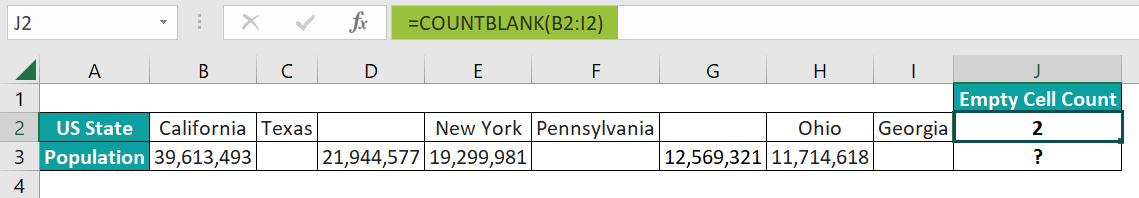2. Drag the formula from cell J2 to J3 using the fill handle. The output is shown below.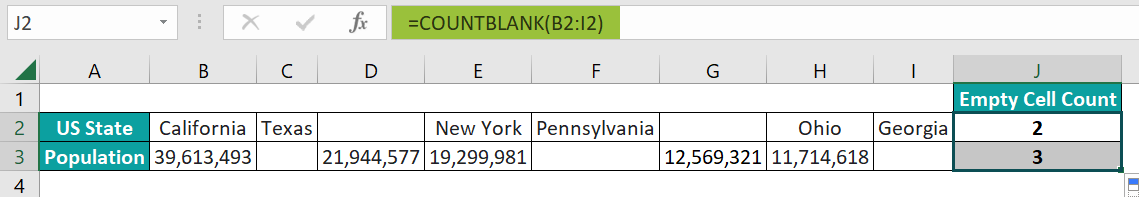[Alternatively, we can choose the target cell J2 and navigate the path FormulasMore FunctionsStatisticalCOUNTBLANK to apply the function in the target cell.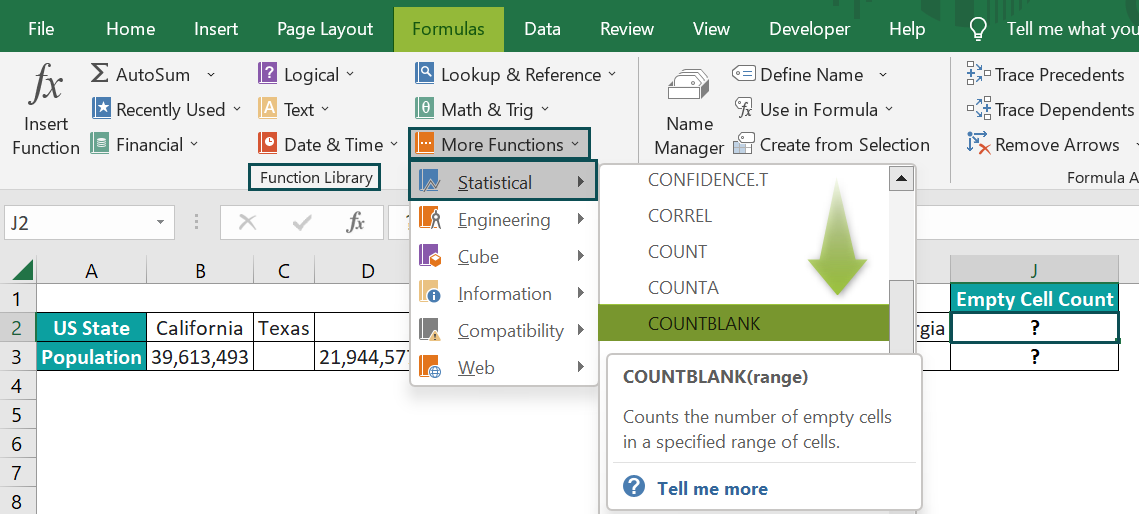The above step will open the Function Arguments window, where we must enter the required cell range as the range argument.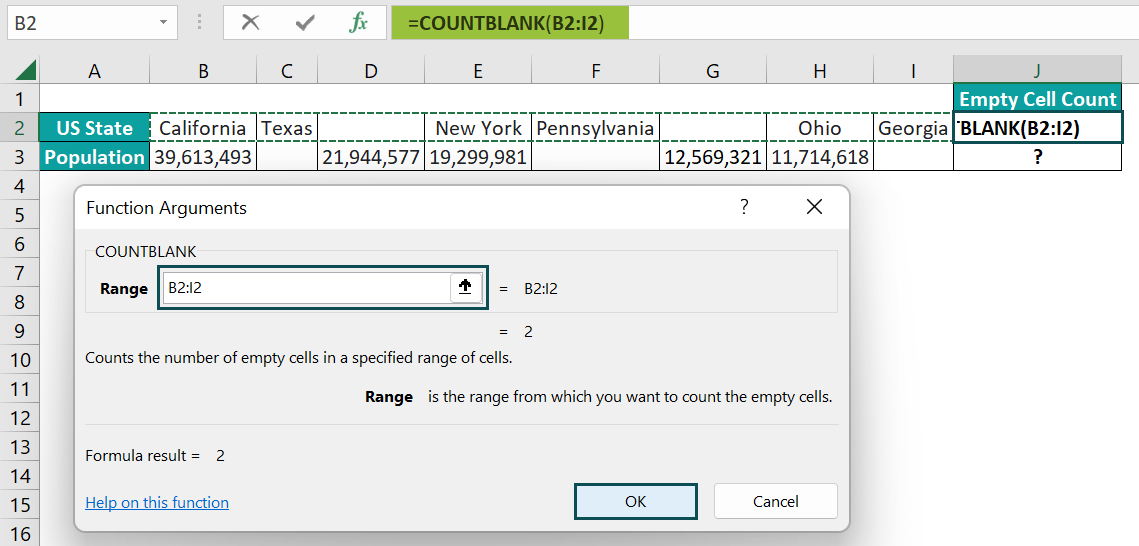And on clicking OK, the window closes, and we can view the COUNTBLANK() output in the target cell J2.]

[Output Observation: The cells C3, F3, and I3 are empty in row 3. And hence the target cell J3 formula returns the total count of blank cells in the given cell range B3:I3 as 3.]

### Examples

We will understand some advanced scenarios using the COUNTBLANK in Excel examples.

#### Example #1

We will determine the total number of blank cells using the COUNTBLANK function.

The below table contains the day-wise sales details of five products.

The procedure to find the total empty/blank cells using the COUNTBLANK formula is,

Select the target cell B8, enter the formula =COUNTBLANK(A1:F6), and press Enter.

The result is ‘9’, as shown above.

[Output Observation: Cell B8 accepts the table range A1:F6 as the input argument. And it counts all the empty cells in the specified cell range to return the sum of all blank cells as 9.]

#### Example #2

We will highlight the blank cells using Conditional Formatting and COUNTBLANK().

The following table shows a list of students and their Physics scores.

The steps to find the empty cells and highlight them using COUNTBLANK() are,

• Step 1: Select cell range A2:B11 → select the “Home” tab → go to the “Styles” group → click the “Conditional Formatting” option drop-down → select the “New Rule” option, as shown below.

The “New Formatting Rule” window appears.

• Step 2: Select the “Use a formula to determine which cells to format” option from the “Select a Rule Type:” group, as shown below.
• Step 3: Enter the formula =COUNTBLANK(\$A2:\$B2) in the “Format values where this formula is true:” field in the “Edit the Rule Description:” group, and click the “Format” button, as shown.

The “Format Cells” window opens. Now, select the “Fill” tab → choose the desired color from the “Background Color:” group, here, Red → click OK to close the “Format Cells” window.

• Step 4: Click OK to close the New Formatting Rule window.

The cells B3, B8, and B10 and their corresponding row cells, based on the specified cell range in the COUNTBLANK(), are highlighted because of the Conditional Formatting feature, as shown below.

#### Example #3

We will check if the data is complete, with 30 entries containing the data of orders delivered by each branch office every month. And if all 30 entries do not contain data, the requirement is to count the total empty cells using the COUNTBLANK(), COUNTA(), and IF excel functions.

The first table in the below image contains a firm’s 6-months orders delivered data across five branch offices.

The steps to use the COUNTA(), IF() and COUNTBLANK() functions to find the empty cells are,

• Step 1: Select cell B9, enter the formula =COUNTA(B2:G6), and press Enter. The result is ‘17’, as shown below.
• Step 2: Select cell B10, enter the IF() with COUNTBLANK() formula =IF(B8=B9,”Nil”,COUNTBLANK(B2:G6)), and press Enter. The result is ‘13’, as shown below.

[Output Observation: First, the COUNTA() in cell B9 returns the number of non-empty cells in the specified cell range B2:G6 as 17. Next, the IF condition in cell B10 checks if the total entries (default) given in cell B8 equals the total entries count the COUNTA() returned in cell B9. As the IF condition does not hold, the FALSE value gets executed, and thus, the COUNTBLANK() returns the total blank cells in the given cell range B2:G6 as 13.]

### Important Things To Note

• The COUNTBLANK in Excel does not consider cells containing numeric values, texts, logical values, error values, zero, or space characters as empty cells.
• The function counts cells containing formulas that return an empty string (“”) as the output.
• Since we have many functions in the COUNT group, we must choose the right function, especially while entering the formula manually. If not, we will get a “#NAME?” error.

1. Where is the COUNTBLANK function in Excel?

The COUNTBLANK function in Excel is in the Formulas tab. First, select the target cell and click FormulasMore FunctionsStatisticalCOUNTBLANK, as shown below.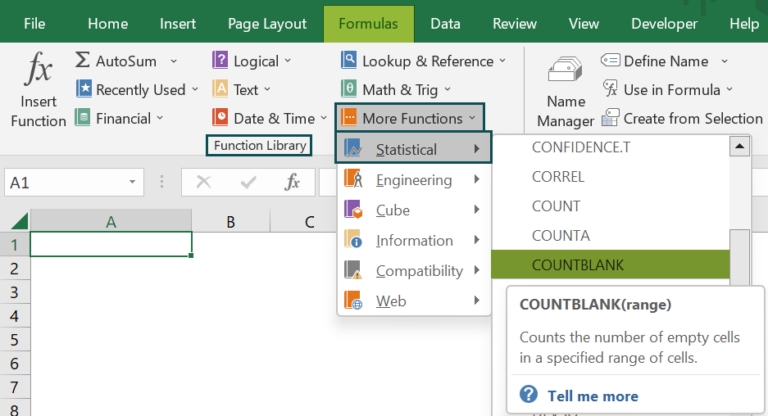2. How to apply the COUNTBLANK function in Excel VBA?

We can apply the COUNTBLANK function in Excel VBA using the following method:

Application.WorksheetFunction.CountBlank(range)

The range argument in the above method is the COUNTBLANK() argument explained in the COUNTBLANK() Excel Formula section.

Let’s consider an example that explains how to apply the COUNTBLANK function in Excel VBA to calculate the total number of blank cells.

The below table contains a list of values and their descriptions.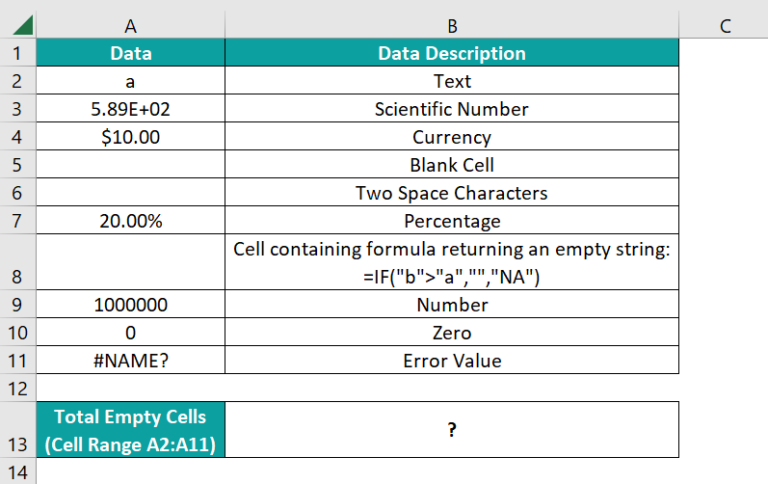The steps to find blank cells using the COUNTBLANK method in Excel VBA are,

• 1: While the worksheet containing the above table is open, open the VBA Editor using Alt + F11.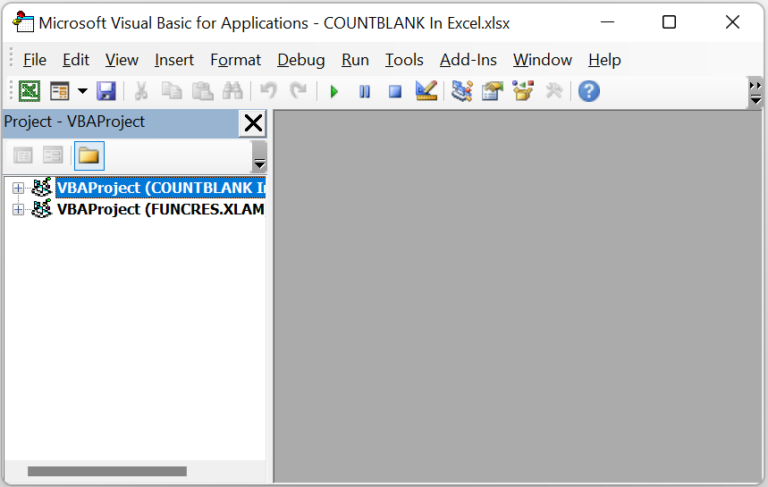• 2: Choose the required VBAProject and select the Module option under the Insert tab to access a new module window, Module1.• 3: Enter the VBA code, as shown in the image below, to apply the COUNTBLANK function in the required target cell.

Sub COUNTBLANK_fn()

Dim ws As Worksheet
Set ws = Worksheets(“FAQ_2”)
ws.Range(“B13”) = Application.WorksheetFunction.CountBlank(ws.Range(“A2:A11”))

End Sub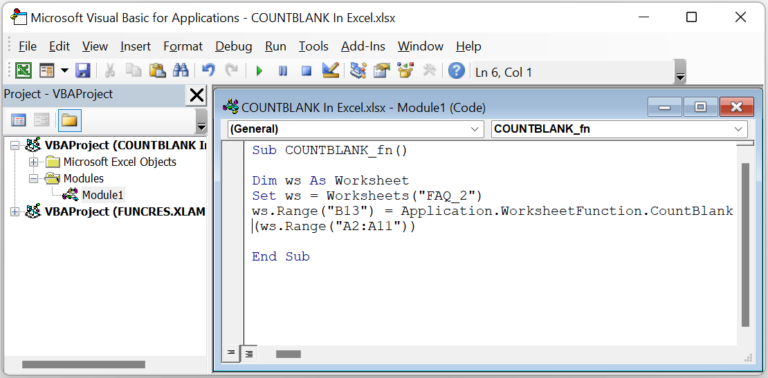• 4: Execute the entered code using the Run Sub/UserForm icon from the top menu.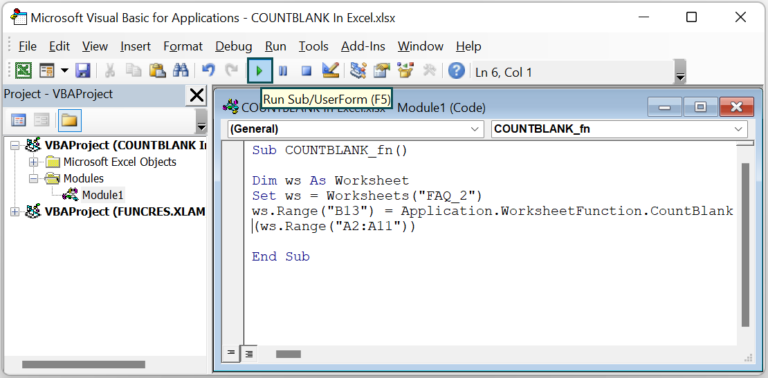• 5: Open the active worksheet to view the total count of empty cells in cell B13.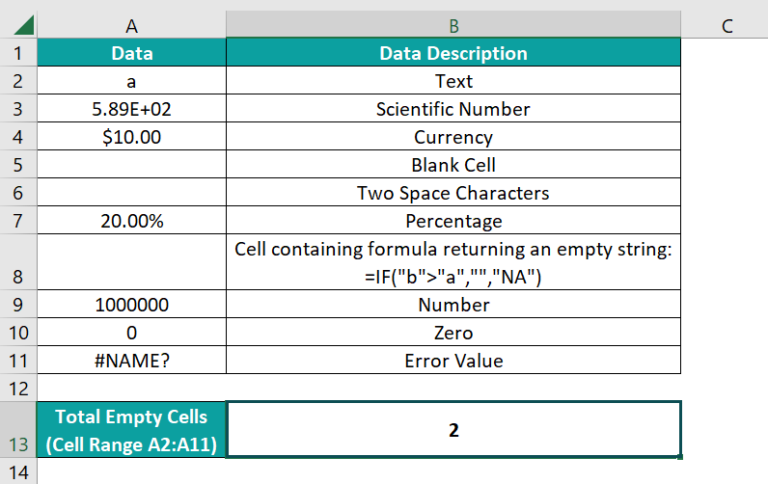[Output Observation: The COUNTBLANK method in the above code accepts the cell range A2:A11 as the input. The function counts the blank cells in the given range. And as cells A5 and A8 count as empty cells, it returns 2 as the output in cell B13.
In the above example, cell A6 does not count as a blank cell, as it contains two space characters.]

3. How can I calculate the total count of blank columns in Excel?

We can calculate the total count of blank columns using the COUNTBLANK, COUNTIF, and ROWS functions.

Consider the following example.
The below table contains the weekly sales report for a set of items.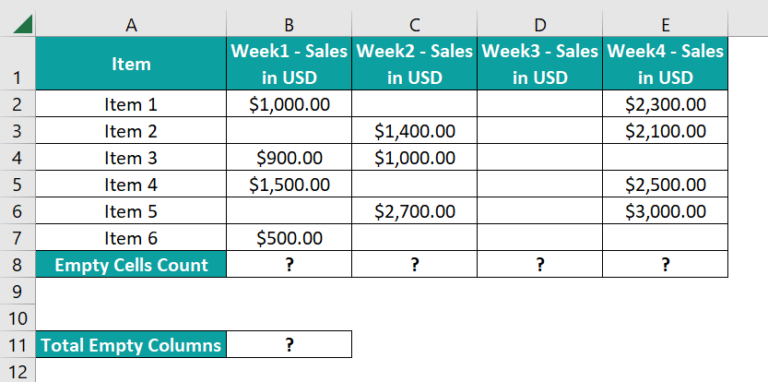The steps to find total empty cells using the COUNTBLANK, COUNTIF, and ROWS functions are,

• 1: Select cell B8, enter the formula =COUNTBLANK(B2:B7), and press Enter. The result is ‘2’, as shown below.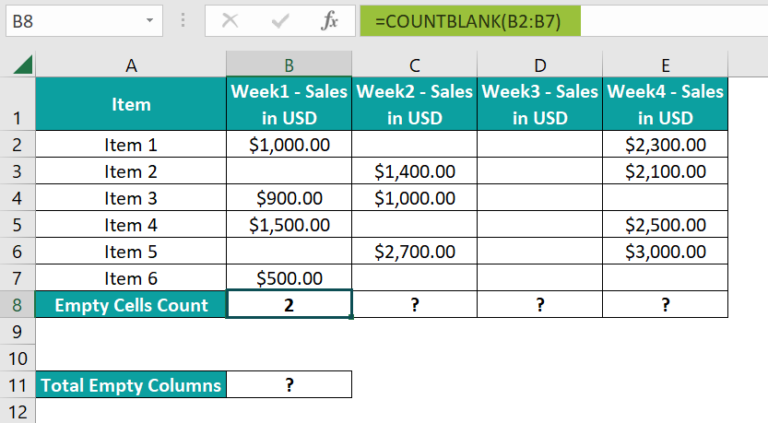• 2: Drag the formula from cell B8 to E8, horizontally, using the fill handle.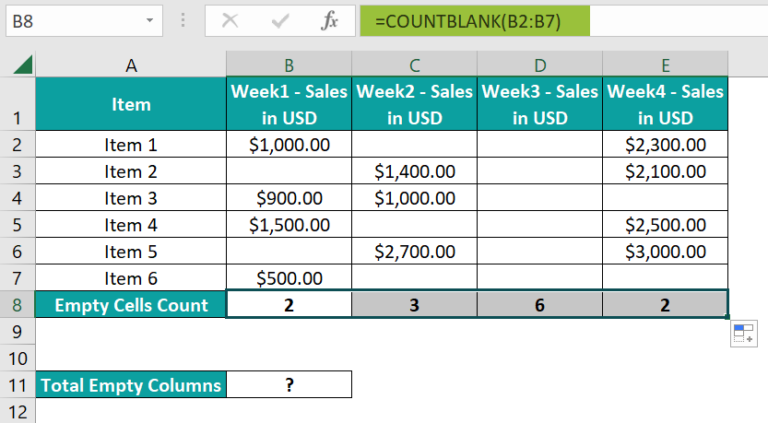• 3: Select cell B11, enter the formula =COUNTIF(B8:E8, ROWS(B2:B7)), and press Enter.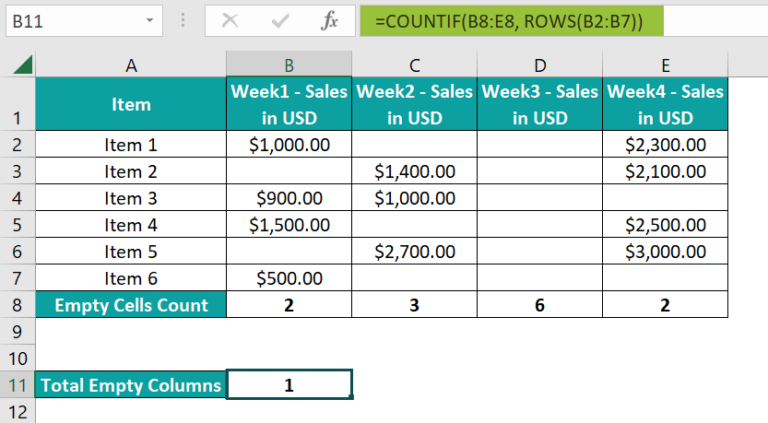The result is ‘2’, as shown below.

[Output Observation: First, the COUNTBLANK functions in cells B8:E8 return the total count of blank cells in each column.
Next, the ROWS() inside the COUNTIF() returns the total rows count in the specified cell range as 6. Finally, the COUNTIF() checks for criteria 6 in the cell range B8:E8. And as only the cell D8 value satisfies the given criteria, the COUNTIF() returns 1 as the total empty columns in the cell range B2:E7.
Thus, the COUNTBLANK() return value helps find a blank column. And the COUNTIF() uses this data to find the sum of all empty columns in a given cell range.]